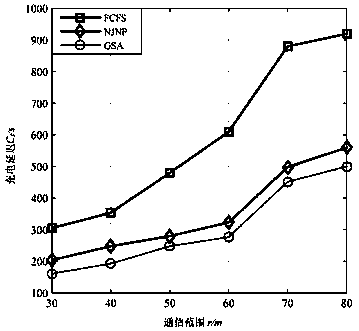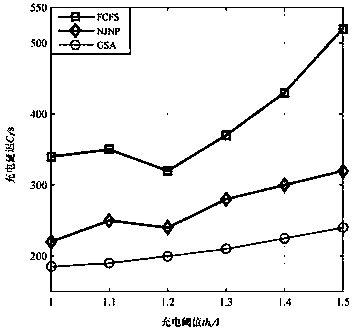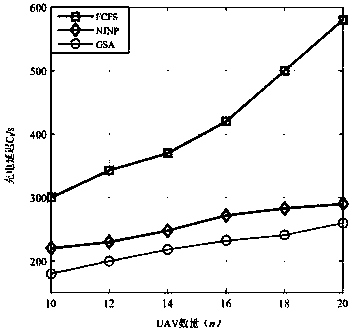# Laser-powered UAV cluster charging scheduling method

## A scheduling method and laser energy supply technology, applied in current collectors, charging stations for mobile device charging, electric vehicles, etc., can solve problems such as relatively few researches on wireless laser charging for drones, reduce charging delay time, guarantee Survival rate, effect of increasing time

Active Publication Date: 2019-10-01
CHINA THREE GORGES UNIV
3 Cites 2 Cited by

## AI-Extracted Technical Summary

### Problems solved by technology

 At present, most of the research on UAV laser energy supply and charging technology is based on the research on the laser wireless ...
the structure of the environmentally friendly knitted fabric provided by the present invention; figure 2 Flow chart of the yarn wrapping machine for environmentally friendly knitted fabrics and storage devices; image 3 Is the parameter map of the yarn covering machine
View more

## Abstract

The invention provides a laser-powered UAV (unmanned aerial vehicle) cluster charging scheduling method. The method comprises a step of acquiring data information of a laser wireless charging networkand establishing a charging charging mathematical model, a step of obtaining initialization data by using a gravity search algorithm according to the charging charging mathematical model and calculating and obtaining a corresponding charging plan fitness function value, a fitness function is a UAV charging delay time C1 which represents an average time taken by a laser charging station to providecharging service for each UAV, and a step of calculating a fitness function value of each charging request queue by the gravity search algorithm to obtain the fitness function value fit(t) of each charging request, finding a best fitness function value b(t) and a worst fitness function value w(t) through comparison, comparing a fitness function value obtained in each round with the best fitness function value b(t) and outputting a charging time sequence table. According to the method of the invention, the time of the tasks of the UAVs can be effectively increased, and at the same time, the survival rate of each UAV in the system can be ensured to the utmost extent.

Application Domain

Technology Topic

## Image

•••## Examples

• Experimental program(1)

### Example Embodiment

 A clustered charging scheduling method for laser-powered drones, the specific steps include:
 1. Establish a laser wireless charging network model for drones:
 Such as image 3 As shown, a laser wireless charging network model includes a set of randomly deployed rechargeable UAVs, and a laser charging station LC that can supplement the energy of UAVs in the charging network area. UAVs in the charging network have the same initial energy, and their energy consumption rate is fixed. However, in a certain period of time, because their states are different, their energy consumption is different from each other. LC can only charge one UAV at a time, and it has enough energy to charge all UAVs in the network. The received power of each UAV is obtained by formula (1).
 2. Charging scheduling algorithm based on gravity search algorithm:
 (1) Fitness function:
 In the charging schedule, the charging request queue is expressed as follows:

 Among them, w is the total number of charging requests in the request queue, It represents the position vector of the dth UAV in the i-th charging request.
 In the gravitational search algorithm, the fitness function value can indirectly calculate the inertial mass of the particle. The larger the inertial mass of the particle, the stronger the attraction and the slower the movement, indicating that the optimization problem has a better solution. Delay charging time C l Set as fitness function:

 Among them, represents LC vs u i The charging time means that LC is giving u i The time to charge all charging requests before charging. w represents the total number of drones in the network.
 (2) Flow of charging scheduling algorithm:
 ①: Use formula (5) to calculate the fitness value of each round of charging request, and calculate the quality value M of each UAV in the algorithm according to each fitness function value of formula (7) i.

 Indicates the best fitness function value in each round of charging, Represents the worst fitness value, fit i (t) represents the fitness value of the i-th UAV at this time, m j (t) represents the mass of the jth UAV. According to Newton’s second law, M ai Represents the active gravitational mass, M pi Represents passive gravitational mass, M ii Represents inertial mass, and: M ai =M pi =M ii =M i
 ②: According to formula (8), calculate the single UAV x at the same moment j For a specific drone x i The force.

 Among them, G(t) represents the gravitational constant at time t, x i The passive gravitational mass is M pi (t) means x i The active gravitational mass is M aj (t) means x i And x j Use R for Euclidean distance between ij (t) means that ε is a very small constant.
 Represents the position of the i-th UAV during the d-th round of charging request, Represents the position of the j-th UAV during the d-th round of charging request.
 The gravitational constant G(t) can be expressed as:

 Among them, G 0 Represents the initial value of the gravity constant, β represents the control parameter value, which is a constant. In general, the value of β is 20, t max Indicates the maximum number of iterations.
 The total force of other drones received by drones in the network is: rand j Is a random number between [0,1], K best From m to 1 decreases linearly with time, so that at the end of the algorithm, only the particle with the best solution in the search space acts on other particles.
 Particle x i The acceleration at time t in dimension d can be expressed as: X in dimension d i The speed and position update can be expressed as:
 3) Use the gravitational search algorithm to continuously update the fitness value, position vector, speed and acceleration of the UAV charged in each round of the network. When the fitness function value of a certain UAV calculated in the algorithm is less than the previous rounds of the algorithm When the best fitness function value is stored in, output the corresponding charging request queue x i.
 The detailed description of the algorithm is as follows:
 Input: the number of charging request queues m, the collection of charging request queues x i , The maximum number of iterations t max ,unmanned
 Machine operating range S
 Initialize the total charge request queue m and initialize the variable set C fit , Used to store fitness value, initial
 A position vector set P is used to store the position vector of the output charging request queue.
 for t=1 to t max , Perform the operation:
 Calculate the fitness value of all drones in the charging request queue fit(t)
 Update the inertial mass M(t) and gravitational constant G(t) of the drone in the charging request queue, the best adaptation
 Degree value b(t), worst fitness value w(t)
 if C fit b(t) is executed:
 Update P, C fit
 end if
 Calculate the total force exerted on each drone
 Calculate the acceleration and speed of all charging request queues
 Update the position vector of all charging request queues
 end for
 According to the charging request queue obtained by the algorithm, the charging plan sequence table is output.
 3. The output of the charging time sequence table
 When the algorithm outputs a set of charging queues, the drones in this queue need to be charged in order, that is, each drone in the queue is sorted. Introduce a random variable z to change the elements in each charging request queue Use a random number z to initialize (z∈[-1,1]) assignment, and then arrange the value of each element in ascending order. The smallest element value will appear in the first position of the schedule, and the second smallest will appear In the second position of the scheduling, and so on, in the end, each round will be based on the charging request queue x i Get a charging request sequence table. The laser charging station LC will charge the drone in an orderly manner according to this charging sequence.
 image 3 It is a graph of the simulation and comparison experiment results of charging delay time in different communication ranges provided by the implementation of the present invention. From image 3 It can be seen that when the number of drones and the charging threshold remain unchanged, the charging delay increases as the communication range of the drone increases, because the energy consumption is proportional to the communication range of the drone, thereby increasing The number of charging requests. For different UAV communication ranges, the charging scheme based on the Gravity Search Algorithm (GSA) has the smallest charging delay compared to the first come first served (FCFS) algorithm and the nearest first served (NJAP) algorithm. The fitness function of the algorithm considers space and time factors to calculate the charging delay, so the obtained charging delay time is optimal.
 Figure 4 It is a graph of the simulation comparison experiment results of charging delay time in different numbers of drone groups provided by the implementation of the present invention. It can be seen from Figure 4 that when the UAV communication range and charging threshold remain unchanged, if the number of UAVs increases, the arrival rate of the charging request will increase accordingly, thereby increasing the charging delay. At the same time, among the three algorithms, the charging scheme based on the gravity search algorithm has the smallest charging waiting time, and the fluctuation of the charging waiting time is also the smallest.
 Figure 5 It is a graph of the simulation comparison experiment results of charging delay time under different charging thresholds provided by the implementation of the present invention. From Figure 5 It can be seen that when the UAV communication range and quantity remain unchanged, under different charging thresholds, similar to the previous two cases, among the three algorithms, the gravity search algorithm still maintains the minimum charging delay time.
the structure of the environmentally friendly knitted fabric provided by the present invention; figure 2 Flow chart of the yarn wrapping machine for environmentally friendly knitted fabrics and storage devices; image 3 Is the parameter map of the yarn covering machine

## PUM## Description & Claims & Application Information

We can also present the details of the Description, Claims and Application information to help users get a comprehensive understanding of the technical details of the patent, such as background art, summary of invention, brief description of drawings, description of embodiments, and other original content. On the other hand, users can also determine the specific scope of protection of the technology through the list of claims; as well as understand the changes in the life cycle of the technology with the presentation of the patent timeline. Login to view more.
the structure of the environmentally friendly knitted fabric provided by the present invention; figure 2 Flow chart of the yarn wrapping machine for environmentally friendly knitted fabrics and storage devices; image 3 Is the parameter map of the yarn covering machine

## Functional Masticatory Material, Method Of Producing The Same And Method Of Using The Same

InactiveUS20090169682A1Increase the number ofExtension of timeMilk preparationBaking mixturesMasticationChemistry
Owner:MEIJI PHARMA

Owner:AUDI AG

## Smart emergency control system

Owner:ZDST COMM TECH CO LTD

## Refillable Pet Treat

InactiveUS20130000564A1Extension of timeCost of treatment can be reducedAnimal feeding devicesOther apparatusEngineeringSolid surface
Owner:BECKHART GORDON HAGGOTT +1

Owner:JU JINGWAN

## Classification and recommendation of technical efficacy words

• Extension of time

## Casing strings and methods of using such strings in subterranean cementing operations

Owner:HALLIBURTON ENERGY SERVICES INC

## Device and system for implanting polyaxial bone fasteners

Owner:ZIMMER SPINE INC

## Rupture-resistant compliant radiopaque catheter balloon and methods for use of same in an intravascular surgical procedure

Owner:VECTOR CORP

## Themed amusement river ride system

InactiveUS20100160054A1Extension of timeReducing amount of required amountRussian swingsGreat wheelsPower stationEngineering
Owner:WATER RIDE CONCEPTS

## Method and apparatus for controlling fluid movement in a microfluidic system

Owner:PETITHORY HENRY

## People also interested in

Who we serve
• R&D Engineer
• R&D Manager
• IP Professional
Why Eureka